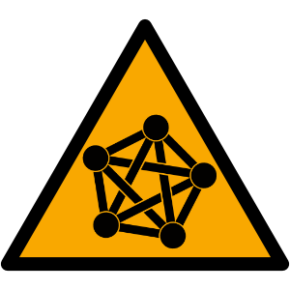samw
/
iso7010-a-day
You cannot select more than 25 topics Topics must start with a letter or number, can include dashes ('-') and can be up to 35 characters long.

#### 31 lines 656 B TOML Raw Permalink Blame History

 ```[package] ``` ```name = "iso7010_a_day" ``` ```description = "A silly twitter bot" ``` ```version = "0.2.0" ``` ```edition = "2021" ``` ``` ``` ```[dependencies] ``` ```reqwest = { version = "0.11", features = ["json", "multipart"]} ``` ```serde_json = "*" ``` ```serde = { version = "1", features = ["derive"] } ``` ```scraper = "*" ``` ```rand = "*" ``` ```resvg = "*" ``` ```oauth1 = "*" ``` ```clap = { version = "*", features = ["derive"] } ``` ```webbrowser = "*" ``` ```#webbrowser = { path = "../webbrowser-rs" } ``` ```itertools = "*" ``` ```tracing = "0.1.36" ``` ```tracing-subscriber = "0.3.15" ``` ```regex = "1.6.0" ``` ```image = "0.24.3" ``` ```viuer = "0.6.1" ``` ```url = { version = "2.3.1", features = ["serde"] } ``` ```megalodon = "0.10" ``` ```tokio = "*" ``` ```futures-util = "*" ``` ``` ``` ```[build-dependencies] ``` ```toml = "*" ```